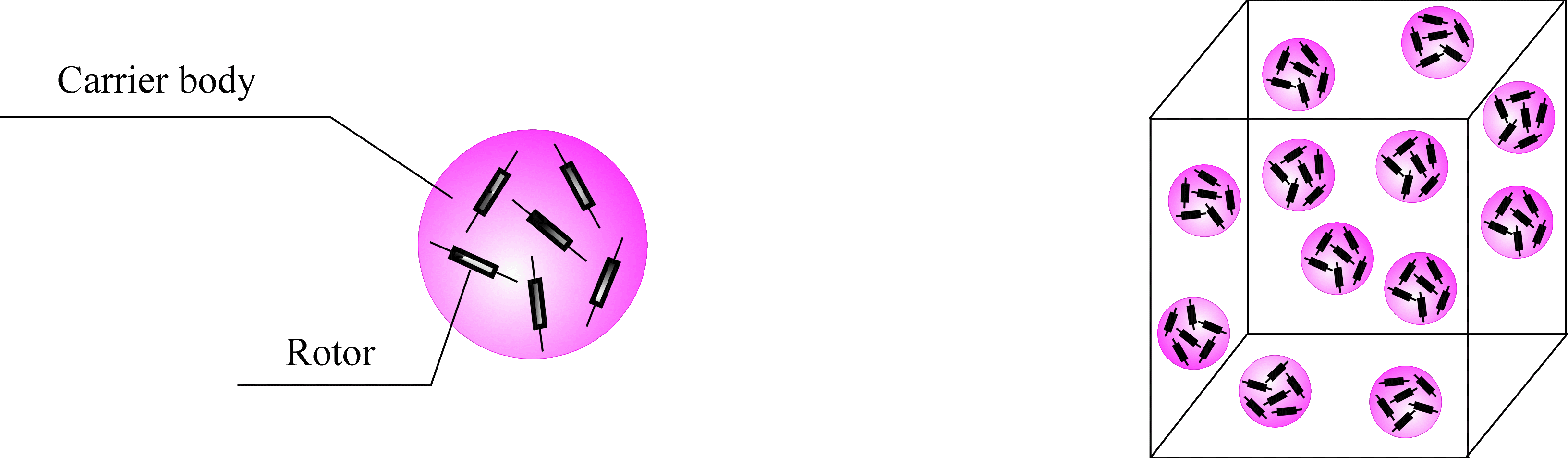Elena A. Ivanova St. Petersburg State Polytechnical UniversityMulti-spin particles and Zhilin's continuum

Multi-spin particles in continuum mechanics

The multi-spin particle is the complex object (see Figure) consisting of the carrier body and N rotors. Suppose that the carrier body and rotors are the infinitesimal rigid bodies. The carrier body has an arbitrary tensor of inertia whereas the rotors are the axisymmetric rigid bodies. The axes of symmetry of the rotors are fixed with respect to the carrier body. The rotors cannot translate relative to the carrier body, and they can rotate independently of rotation of the carrier body only about their axes of symmetry. Each point of the material medium (see Figure) consisting of such particles has N+6 degrees of freedom. For the first time the continuum of multi-spin particles was introduced by P.A.Zhilin in order to use this continuum as a mechanical model of the electromagnetic field. The system of equations describing the continuum of multi-spin particles, in addition to the standard continuum mechanics equations, includes N scalar equations describing the rotation of rotors relative to the carrier bodies. The fact that the energy balance equation contains the terms depending on the velocity of rotors rotations relative to carrier body is an important feature that deserves attention. After the reduction of energy balance equation to the standard for continuum mechanics form, these terms should be interpreted as the energy supply due to the motion of internal structure. Thus, the continuum of multi-spin particle has the ability to store the internal energy which is not the energy of deformation.Multi-spin particle and a representative volume in continuum consisting of multi-spin particles

Continuum of multi-spin particles as a model of electromagnetic field

Substantiating the need for a mechanical model of the electromagnetic field P.A.Zhilin wrote (see "Rational Continuum Mechanics", p. 312): "Suppose that the classical Maxwell equations do not satisfy us for some reason and they should be changed in some way. This is not hypothetical assumption since it is known that the classical equations do not allow us to construct a consistent theory of the atom. Therefore, changes are needed, but what exactly needs to be changed? The classical equations do not answer this question. The presence of a mechanical interpretation gives the direction of refinements and also shows the urgent need of the refinements." P.A.Zhilin was convinced that the main type of motion in the electromagnetic field is spin (rotational) motion, i.e. the electromagnetic field is a medium which consists of fast rotating particles. It is through the energy of rotating particles the electromagnetic field stores its proper energy. The classical model of the electromagnetic field described by Maxwell's equations does not possess this property. Note that the electromagnetic field in Zhilin's interpretation differs from the traditional concepts. To clarify the aforesaid we give one more quote from the book "Rational Continuum Mechanics" (p. 281): "It is important to note that the electromagnetic field in itself has no relation to what is called a charge in physics. This is a strong contrast from the point of view taken in physics, in accordance to which the electromagnetic field is generated by the charges. However, the charged bodies are making disturbances in the electromagnetic field. If these disturbances are called electromagnetic field, then the terminological differences with the physics disappear. We prefer to use the term "electromagnetic field" for the medium itself, and not for the perturbation in it." The description of P.A.Zhilin's theory can be found in the sixth chapter of his book:

• Zhilin P. A. Rational Continuum Mechanics. Politechnic University Publishing House, St. Petersburg. 2012. 584 p. (In Russian)